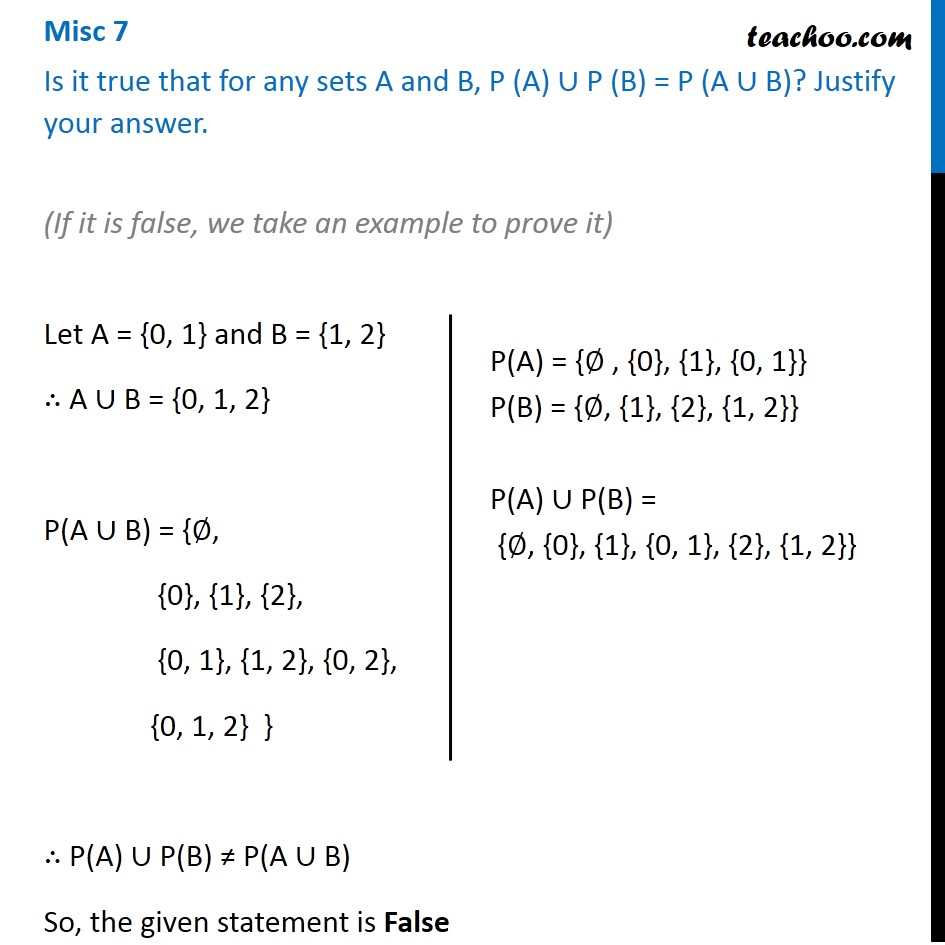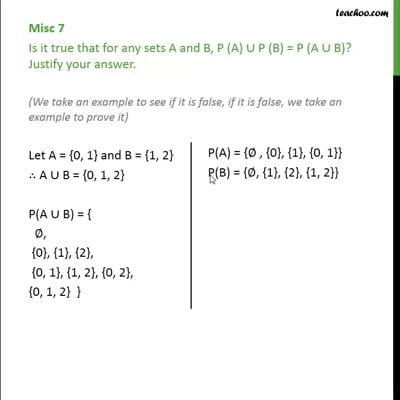Proof - where properties of sets cant be applied,using element

Chapter 1 Class 11 Sets
Concept wiseThis video is only available for Teachoo black users

Introducing your new favourite teacher - Teachoo Black, at only ₹83 per month

### Transcript

Misc 7 Is it true that for any sets A and B, P (A) ∪ P (B) = P (A ∪ B)? Justify your answer. (If it is false, we take an example to prove it) Let A = {0, 1} and B = {1, 2} ∴ A ∪ B = {0, 1, 2} P(A ∪ B) = {∅, {0}, {1}, {2}, {0, 1}, {1, 2}, {0, 2}, {0, 1, 2} } ∴ P(A) ∪ P(B) ≠ P(A ∪ B) So, the given statement is False P(A) = {∅ , {0}, {1}, {0, 1}} P(B) = {∅, {1}, {2}, {1, 2}} P(A) ∪ P(B) = {∅, {0}, {1}, {0, 1}, {2}, {1, 2}}